# Forecasting Stock Returns Using ARIMA Model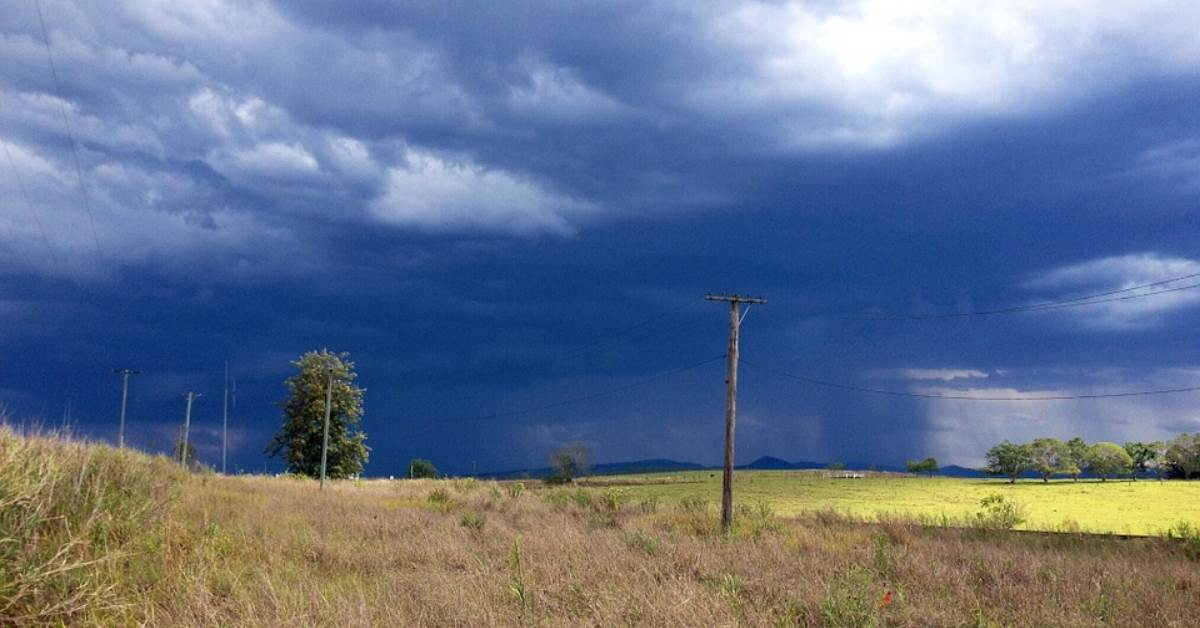Stock price prediction is very difficult, especially about the future”. Many of you must have come across this famous quote by Neils Bohr, a Danish physicist. Stock price prediction is the theme of this blog post. In this post, we will cover the popular ARIMA forecasting model to predict returns on a stock and demonstrate a step-by-step process of ARIMA modeling using R programming.

### What is a forecasting model in Time Series?

Forecasting involves predicting values for a variable using its historical data points or it can also involve predicting the change in one variable given the change in the value of another variable. Forecasting approaches are primarily categorized into qualitative forecasting and quantitative forecasting. Time series forecasting falls under the category of quantitative forecasting wherein statistical principles and concepts are applied to a given historical data of a variable to forecast the future values of the same variable. Some time series forecasting techniques used include:
• Autoregressive Models (AR)
• Moving Average Models (MA)
• Seasonal Regression Models
• Distributed Lags Models

### What is Autoregressive Integrated Moving Average (ARIMA) Model?

ARIMA stands for Autoregressive Integrated Moving Average. ARIMA is also known as Box-Jenkins approach. Box and Jenkins claimed that non-stationary data can be made stationary by differencing the series, Yt. The general model for Yt is written as,

Yt1Yt1 2Yt2...ϕpYtpt + θ1ϵt1+ θ2ϵt2 +...θqϵtq

Where Yt is the differenced time series value, ϕ and θ are unknown parameters and ϵ are independent identically distributed error terms with zero mean. Here, Yt is expressed in terms of its past values and the current and past values of error terms.

The ARIMA model combines three basic methods:

• AutoRegression (AR) – In auto-regression, the values of a given time series data are regressed on their own lagged values, which is indicated by the “p” value in the ARIMA model.
• Differencing (I-for Integrated) – This involves differencing the time series data to remove the trend and convert a non-stationary time series to a stationary one. This is indicated by the “d” value in the ARIMA model. If d = 1, it looks at the difference between two-time series entries, if d = 2 it looks at the differences of the differences obtained at d =1, and so forth.
• Moving Average (MA) – The moving average nature of the ARIMA model is represented by the “q” value which is the number of lagged values of the error term.
This model is called Autoregressive Integrated Moving Average or ARIMA(p,d,q) of Yt.  We will follow the steps enumerated below to build our model.

Step 1: Testing and Ensuring Stationarity

To model a time series with the Box-Jenkins approach, the series has to be stationary. A stationary time series means a time series without trend, one having a constant mean and variance over time, which makes it easy for predicting values.

Testing for stationarity - We test for stationarity using the Augmented Dickey-Fuller unit root test. The p-value resulting from the ADF test has to be less than 0.05 or 5% for a time series to be stationary. If the p-value is greater than 0.05 or 5%, you conclude that the time series has a unit root which means that it is a non-stationary process.

Differencing – To convert a non-stationary process to a stationary process, we apply the differencing method. Differencing a time series means finding the differences between consecutive values of a time series data. The differenced values form a new time series dataset which can be tested to uncover new correlations or other interesting statistical properties.

We can apply the differencing method consecutively more than once, giving rise to the "first differences", "second order differences", etc.

We apply the appropriate differencing order (d) to make a time series stationary before we can proceed to the next step.

Step 2: Identification of p and q

In this step, we identify the appropriate order of Autoregressive (AR) and Moving average (MA) processes by using the Autocorrelation function (ACF) and Partial Autocorrelation function (PACF).  Please refer to our blog, “Starting out with Time Series” for an explanation of ACF and PACF functions.

Identifying the p order of AR model

For AR models, the ACF will dampen exponentially and the PACF will be used to identify the order (p) of the AR model. If we have one significant spike at lag 1 on the PACF, then we have an AR model of the order 1, i.e. AR(1). If we have significant spikes at lag 1, 2, and 3 on the PACF, then we have an AR model of the order 3, i.e. AR(3).

Identifying the q order of MA model

For MA models, the PACF will dampen exponentially and the ACF plot will be used to identify the order of the MA process. If we have one significant spike at lag 1 on the ACF, then we have an MA model of the order 1, i.e. MA(1). If we have significant spikes at lag 1, 2, and 3 on the ACF, then we have an MA model of the order 3, i.e. MA(3).

Step 3: Estimation and Forecasting

Once we have determined the parameters (p,d,q) we estimate the accuracy of the ARIMA model on a training data set and then use the fitted model to forecast the values of the test data set using a forecasting function. In the end, we cross-check whether our forecasted values are in line with the actual values.

### Building ARIMA model using R programming

Now, let us follow the steps explained to build an ARIMA model in R. There are a number of packages available for time series analysis and forecasting. We load the relevant R package for time series analysis and pull the stock data from yahoo finance.
```library(quantmod);library(tseries);
library(timeSeries);library(forecast);library(xts);# Pull data from Yahoo finance
TECHM = getSymbols('TECHM.NS', from='2012-01-01', to='2015-01-01',auto.assign = FALSE)
TECHM = na.omit(TECHM)# Select the relevant close price series
stock_prices = TECHM[,4]```
In the next step, we compute the logarithmic returns of the stock as we want the ARIMA model to forecast the log returns and not the stock price. We also plot the log return series using the plot function.
```# Compute the log returns for the stock
stock = diff(log(stock_prices),lag=1)
stock = stock[!is.na(stock)]# Plot log returns
plot(stock,type='l', main='log returns plot')```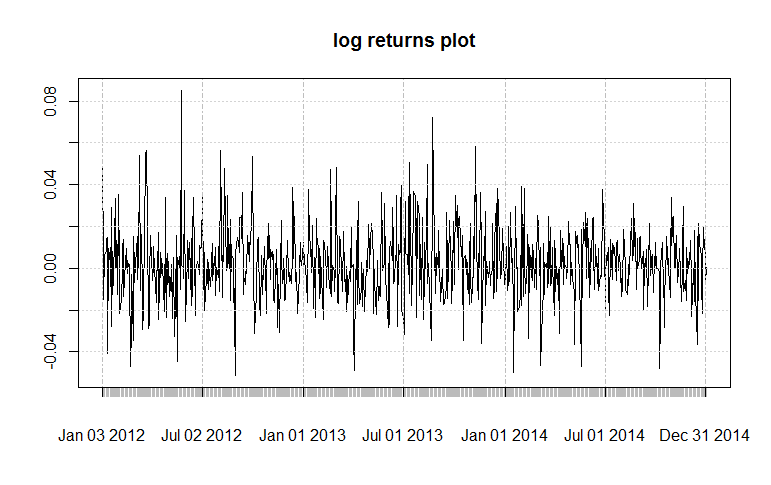Next, we call the ADF test on the returns series data to check for stationarity. The p-value of 0.01 from the ADF test tells us that the series is stationary. If the series were to be non-stationary, we would have first differenced the returns series to make it stationary.

```# Conduct ADF test on log returns series
```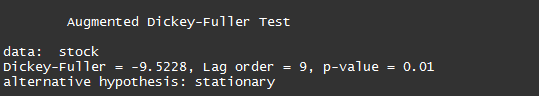In the next step, we fixed a breakpoint which will be used to split the returns dataset in two parts further down the code.

```# Split the dataset in two parts - training and testing
breakpoint = floor(nrow(stock)*(2.9/3))```

We truncate the original returns series till the breakpoint and call the ACF and PACF functions on this truncated series.

```# Apply the ACF and PACF functions
par(mfrow = c(1,1))
acf.stock = acf(stock[c(1:breakpoint),], main='ACF Plot', lag.max=100)
pacf.stock = pacf(stock[c(1:breakpoint),], main='PACF Plot', lag.max=100)```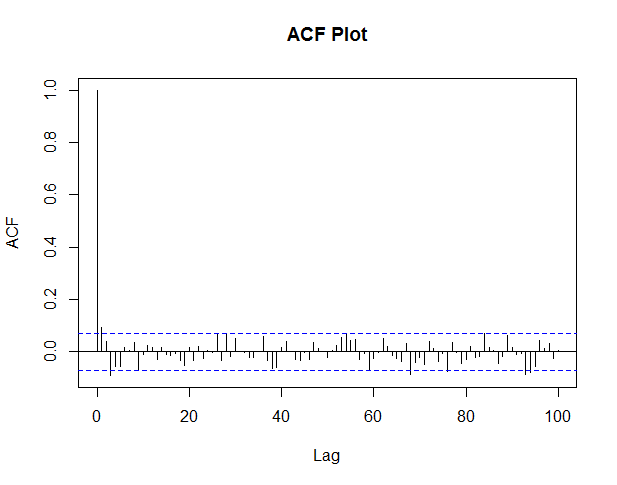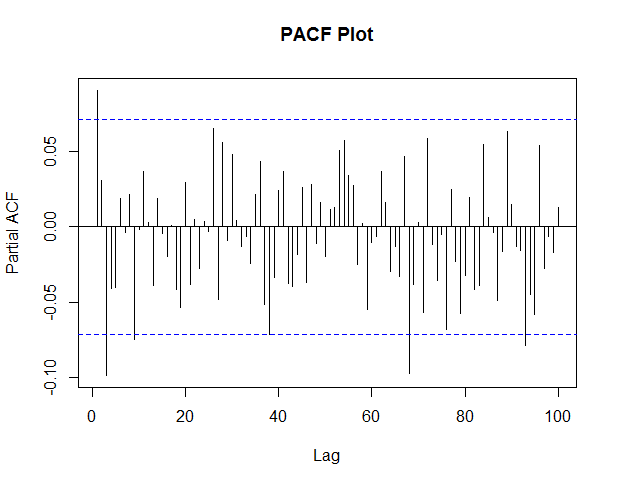We can observe these plots and arrive at the Autoregressive (AR) order and Moving Average (MA) order.

We know that for AR models, the ACF will dampen exponentially and the PACF plot will be used to identify the order (p) of the AR model. For MA models, the PACF will dampen exponentially and the ACF plot will be used to identify the order (q) of the MA model. From these plots let us select AR order = 2 and MA order = 2. Thus, our ARIMA parameters will be (2,0,2).

Our objective is to forecast the entire returns series from breakpoint onwards. We will make use of the For Loop statement in R and within this loop, we will forecast returns for each data point from the test dataset.

In the code given below, we first initialize a series which will store the actual returns and another series to store the forecasted returns.  In the For Loop, we first form the training dataset and the test dataset based on the dynamic breakpoint.

We call the ARIMA function on the training dataset for which the order specified is (2, 0, 2). We use this fitted model to forecast the next data point by using the forecast.Arima function. The function is set at 99% confidence level. One can use the confidence level argument to enhance the model. We will be using the forecasted point estimate from the model. The “h” argument in the forecast function indicates the number of values that we want to forecast, in this case, the next day returns.

We can use the summary function to confirm the results of the ARIMA model are within acceptable limits. In the last part, we append every forecasted return and the actual return to the forecasted returns series and the actual returns series respectively.

```# Initialzing an xts object for Actual log returns
Actual_series = xts(0,as.Date("2014-11-25","%Y-%m-%d"))# Initialzing a dataframe for the forecasted return series
forecasted_series = data.frame(Forecasted = numeric())

for (b in breakpoint:(nrow(stock)-1)) {

stock_train = stock[1:b, ]
stock_test = stock[(b+1):nrow(stock), ]# Summary of the ARIMA model using the determined (p,d,q) parameters
fit = arima(stock_train, order = c(2, 0, 2),include.mean=FALSE)
summary(fit)# plotting a acf plot of the residuals
acf(fit\$residuals,main="Residuals plot")# Forecasting the log returns
arima.forecast = forecast.Arima(fit, h = 1,level=99)
summary(arima.forecast)# plotting the forecast
par(mfrow=c(1,1))
plot(arima.forecast, main = "ARIMA Forecast")# Creating a series of forecasted returns for the forecasted period
forecasted_series = rbind(forecasted_series,arima.forecast\$mean)
colnames(forecasted_series) = c("Forecasted")# Creating a series of actual returns for the forecasted period
Actual_return = stock[(b+1),]
Actual_series = c(Actual_series,xts(Actual_return))
rm(Actual_return)

print(stock_prices[(b+1),])
print(stock_prices[(b+2),])

}```

Before we move to the last part of the code, let us check the results of the ARIMA model for a sample data point from the test dataset.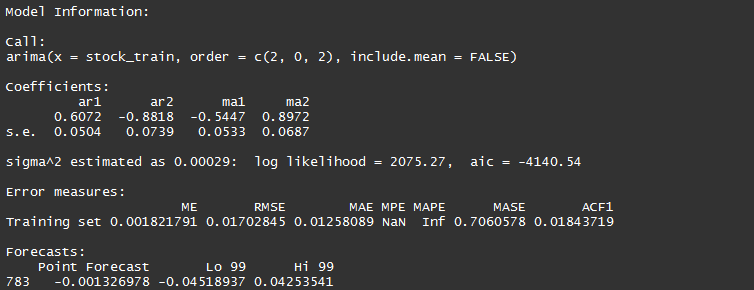From the coefficients obtained, the return equation can be written as:

Yt = 0.6072Y(t-1)  - 0.8818Y(t-2) - 0.5447ε(t-1) + 0.8972ε(t-2)

The standard error is given for the coefficients, and this needs to be within the acceptable limits. The Akaike information criterion (AIC) score is a good indicator of the ARIMA model accuracy. Lower the AIC score better the model. We can also view the ACF plot of the residuals; a good ARIMA model will have its autocorrelations below the threshold limit. The forecasted point return is -0.001326978, which is given in the last row of the output.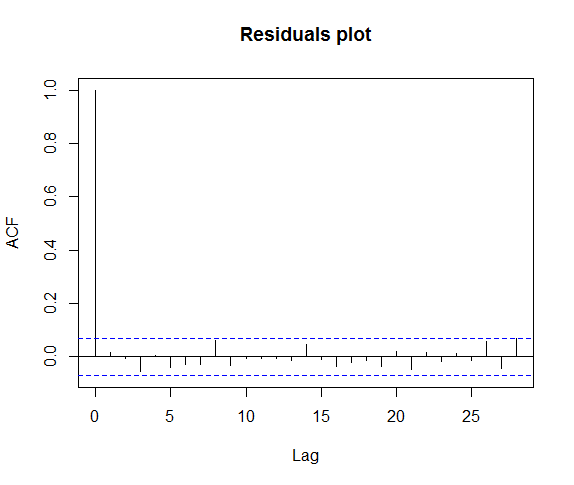Let us check the accuracy of the ARIMA model by comparing the forecasted returns versus the actual returns. The last part of the code computes this accurate information.

```# Adjust the length of the Actual return series
Actual_series = Actual_series[-1]# Create a time series object of the forecasted series
forecasted_series = xts(forecasted_series,index(Actual_series))# Create a plot of the two return series - Actual versus Forecasted
plot(Actual_series,type='l',main='Actual Returns Vs Forecasted Returns')
lines(forecasted_series,lwd=1.5,col='red')
legend('bottomright',c("Actual","Forecasted"),lty=c(1,1),lwd=c(1.5,1.5),col=c('black','red'))# Create a table for the accuracy of the forecast
comparsion = merge(Actual_series,forecasted_series)
comparsion\$Accuracy = sign(comparsion\$Actual_series)==sign(comparsion\$Forecasted)
print(comparsion)# Compute the accuracy percentage metric
Accuracy_percentage = sum(comparsion\$Accuracy == 1)*100/length(comparsion\$Accuracy)
print(Accuracy_percentage)```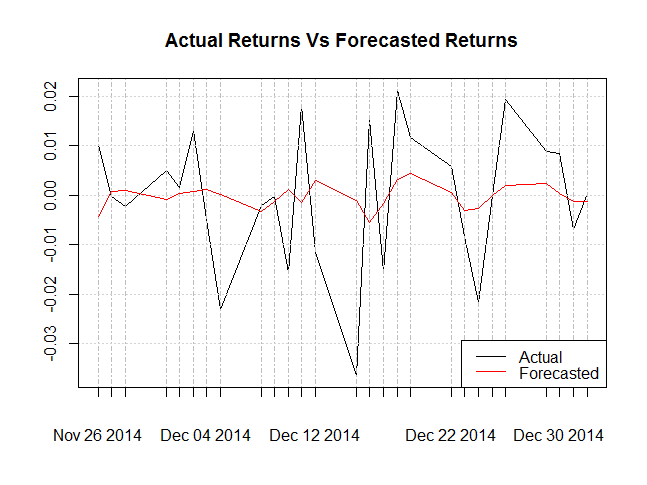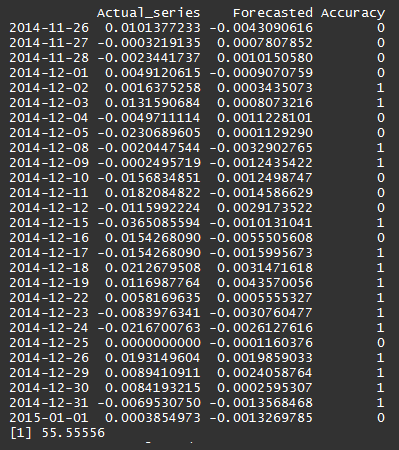If the sign of the forecasted return equals the sign of the actual returns we have assigned it a positive accuracy score. The accuracy percentage of the ARIMA model comes to around 55% which looks like a decent number. One can try running the model for other possible combinations of (p,d,q) or instead use the auto.arima function which selects the best optimal parameters to run the ARIMA model.

### Conclusion

To conclude, in this post we covered the ARIMA model and applied it to forecasting stock price returns using R programming language. We also crossed checked our forecasted results with the actual returns. In our upcoming posts, we will cover other time series forecasting techniques and try them in Python/R programming languages.

### Next Step

Here is a Step by step tutorial for you to implement Predictive Modeling in R for automated trading. You can use predictive model to glance at historical data for algorithmic trading.

Update

We have noticed that some users are facing challenges while downloading the market data from Yahoo and Google Finance platforms. In case you are looking for an alternative source for market data, you can use Quandl for the same.

Disclaimer: All investments and trading in the stock market involve risk. Any decisions to place trades in the financial markets, including trading in stock or options or other financial instruments is a personal decision that should only be made after thorough research, including a personal risk and financial assessment and the engagement of professional assistance to the extent you believe necessary. The trading strategies or related information mentioned in this article is for informational purposes only.